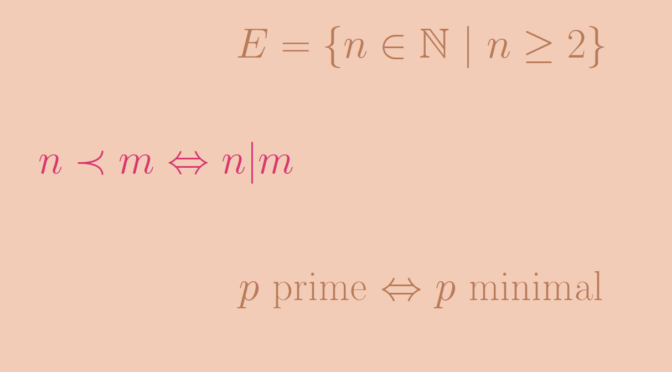# A partially ordered set having multiple minimal elements

Let’s consider a partially ordered set (or poset) $$E$$.

If $$E$$ is totally ordered, $$E$$ has at most one minimal element. If $$E$$ is not totally ordered, $$E$$ can have multiple minimal elements. We provide an example for the set $$E=\{n \in \mathbb N \ | \ n \ge 2\}$$. For two natural numbers $$n$$ and $$m$$, we write $$n|m$$ if $$n$$ divides $$m$$. One easily sees that this yields a partial order.

The minimal elements of $$E$$ are the elements not having divisors, this is the case for all prime numbers $$p \in E$$.

$$E$$ has an infinite number of minimal elements which are the prime numbers.